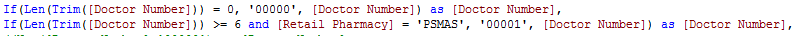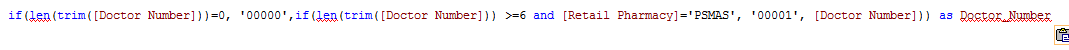# New to QlikView

Discussion board where members can get started with QlikView.

Highlighted
Valued Contributor

## Expression consolidation

Can the following two expressions be combined into one expression:Regards.

Tags (1)
1 Solution

Accepted Solutions
Esteemed Contributor

## Re: Expression consolidation

like this?

=if(len(trim([Doctor Number]))=0, '00000',

if(len(trim([Doctor Number])) >=6 and [Retail Pharmacy]='PSMAS', '00001', [Doctor Number])) as [Doctor Number]

11 Replies
Esteemed Contributor

## Re: Expression consolidation

like this?

=if(len(trim([Doctor Number]))=0, '00000',

if(len(trim([Doctor Number])) >=6 and [Retail Pharmacy]='PSMAS', '00001', [Doctor Number])) as [Doctor Number]MVP & Luminary

## Re: Expression consolidation

HI,

Try like this

=If(Len(Trim([Doctor Number]))=0, '00000',

If(Len(Trim([Doctor Number])) >=6 AND[Retail Pharmacy]='PSMAS', '00001', [Doctor Number])) AS [Doctor Number]

Valued Contributor

## Re: Expression consolidation

Many thanks Bairaj, i will try it out and advise outcome

Regards

Valued Contributor

## Re: Expression consolidation

Thanks Jagan, i am trying this out and will let you know outcome

Regards.

Honored Contributor III

## Re: Expression consolidation

Hi Chris,

This should work:Thanks,

AS

Valued Contributor

## Re: Expression consolidation

Amit may thanks i am trying this outMVP

## Re: Expression consolidation

Valued Contributor

## Re: Expression consolidation

Hi Balraj

Many thanks that works.  i have problems with using the if(Len(Trim function on its own and want to incorporate it with the NULL function as follows:

if(len(trim([Doctor Number]) = 0 or If(IsNull([Doctor Number])), '00000',  if(len(trim([Doctor Number])) >= 7 and [Retail Pharmacy]='PSMAS', '00001', [Doctor Number])) as [Doctor Number]

There is something wrong with the above expression and i am failing to figure out the error.  Kindly assist

Honored Contributor III

## Re: Expression consolidation

Try:

if(IsNull([Doctor Number]),'00000',if(len(trim([Doctor Number])) >= 7 and [Retail Pharmacy]='PSMAS', '00001', [Doctor Number])) as [Doctor Number]

Thanks,

AS